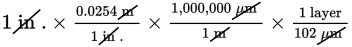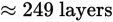# SAT Math Multiple Choice Question 843: Answer and Explanation

### Test Information

Question: 843

3. A typical 3-D printer creates objects by laying down layers of ink one on top of another.The average thickness of a layer depends on the caliber of the printer. For one particular3-D printer, the average thickness of a layer is 102 μm (micrometers). When creatinga 1-inch cube, the printer takes 6 seconds to lay down each layer of ink. Approximatelyhow long should it take this printer to create a 1-inch cube? (There are 1,000,000micrometers in a meter, and 1 inch is approximately equal to 0.0254 meters.)

• A. 12 minutes
• B. 25 minutes
• C. 40 minutes
• D. 1 hour, 6 minutes

Explanation:

B

Difficulty: Hard

Category: Problem Solving and Data Analysis / Rates, Ratios, Proportions, and Percentages

Strategic Advice: When a question involves multiple unit conversions, make a plan to keep things organized. Using the factor-label method (canceling units) also helps.

Getting to the Answer: Think backward: To determine how long it will take to print the cube, you need to know how many layers are required. To determine the number of layers needed, convert micrometers to meters and then meters to inches. Don't forget to line up the conversions so that the units cancel nicely. Start with 1 inch and work your way toward layers:The printer takes 6 seconds to print one layer, so it takes 6 × 249 = 1,494 seconds to print the cube. There are 60 seconds in 1 minute, so it takes 1,494 ÷ 60 = 24.9, or about 25 minutes to print the cube. Choice (B) is correct.